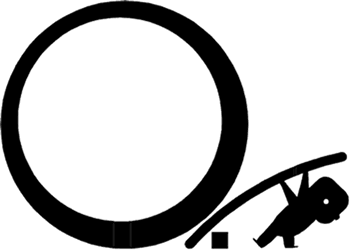In the past, spot forex was only traded in specific amounts called lots, or basically the number of currency units you will buy or sell.

The standard size for a lot is 100,000 units of currency, and now, there are also a mini, micro, and nano lot sizes that are 10,000, 1,000, and 100 units respectively.

Lot Number of Units
Standard 100,000
Mini 10,000
Micro 1,000
Nano 100

As you may already know, the change in currency value relative to another is measured in “pips,” which is a very, very small percentage of a unit of currency’s value.

To take advantage of this minute change in value, you need to trade large amounts of a particular currency in order to see any significant profit or loss.

Let’s assume we will be using a 100,000 unit (standard) lot size. We will now recalculate some examples to see how it affects the pip value.

1. USD/JPY at an exchange rate of 119.80: (.01 / 119.80) x 100,000 = \$8.34 per pip
2. USD/CHF at an exchange rate of 1.4555: (.0001 / 1.4555) x 100,000 = \$6.87 per pip

In cases where the U.S. dollar is not quoted first, the formula is slightly different.

1. EUR/USD at an exchange rate of 1.1930: (.0001 / 1.1930) X 100,000 = 8.38 x 1.1930 = \$9.99734 rounded up will be \$10 per pip
2. GBP/USD at an exchange rate of 1.8040: (.0001 / 1.8040) x 100,000 = 5.54 x 1.8040 = 9.99416 rounded up will be \$10 per pip.

Your broker may have a different convention for calculating pip values relative to lot size but whichever way they do it, they’ll be able to tell you what the pip value is for the currency you are trading is at that particular time.

As the market moves, so will the pip value depending on what currency you are currently trading.

## What the heck is leverage?

You are probably wondering how a small investor like yourself can trade such large amounts of money.

Think of your broker as a bank who basically fronts you \$100,000 to buy currencies.

All the bank asks from you is that you give it \$1,000 as a good faith deposit, which it will hold for you but not necessarily keep.

Sounds too good to be true? This is how forex trading using leverage works.The amount of leverage you use will depend on your broker and what you feel comfortable with.

Typically the broker will require a trade deposit, also known as “account margin” or “initial margin.” Once you have deposited your money you will then be able to trade. The broker will also specify how much they require per position (lot) traded.

For example, if the allowed leverage is 100:1 (or 1% of position required), and you wanted to trade a position worth \$100,000, but you only have \$5,000 in your account.

No problem as your broker would set aside \$1,000 as down payment, or the “margin,” and let you “borrow” the rest.

Of course, any losses or gains will be deducted or added to the remaining cash balance in your account.

The minimum security (margin) for each lot will vary from broker to broker.

In the example above, the broker required a one percent margin. This means that for every \$100,000 traded, the broker wants \$1,000 as a deposit on the position.

## How the heck do I calculate profit and loss?

So now that you know how to calculate pip value and leverage, let’s look at how you calculate your profit or loss.

Let’s buy U.S. dollars and sell Swiss francs.

1. The rate you are quoted is 1.4525 / 1.4530. Because you are buying U.S. dollars you will be working on the “ASK” price of 1.4530, the rate at which traders are prepared to sell.
2. So you buy 1 standard lot (100,000 units) at 1.4530.
3. A few hours later, the price moves to 1.4550 and you decide to close your trade.
4. The new quote for USD/CHF is 1.4550 / 1.4555. Since you initially bought to open the trade, to close the trade, you now must sell in order to close the trade so you must take the “BID” price of 1.4550. The price which traders are prepared to buy at.
5. The difference between 1.4530 and 1.4550 is .0020 or 20 pips.
6. Using our formula from before, we now have (.0001/1.4550) x 100,000 = \$6.87 per pip x 20 pips = \$137.40